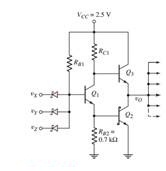+1-415-670-9189
info@expertsmind.com

Get Solution

Determine the values of rb1 and rc1
Course:- Electrical Engineering
Reference No.:- EM131236494

 TweetExpertsmind Rated 4.9 / 5 based on 47215 reviews.
Review Site
Assignment Help >> Electrical Engineering

Consider the modified Schottky TTL NAND gate shown in Figure. The current gain of all transistors is β = 20.

(a) Assume vX = vY = vZ = logic 1 and assume two similar type load circuits are connected to the output. The transistor Q2 is biased in saturation with iB2 = 0.1 mA and iC2 = 0.2 mA. Determine the values of RB1 and RC1.

(b) Using the results of part (a), and assuming vX = 0.4 V and v= vZ = 1.8 V, determine vB1, vB2, vO , and all base and collector currents. Assume two similar type load circuits are connected to the output.

(c) Assume vX = vY = vZ = logic 1 and assume four similar type load circuits are connected to the output. Using the results of part (a), determine vB1, vB2, vO , and all base and collector currents.

(d) Determine the maximum fanout for a low output state.Minimize

Ask Question & Get Answers from Experts
Browse some more (Electrical Engineering) Materials
 Important information about RC Series Circuit, An RC series circuit has a voltage source of 10Vac, a resistor of 10ohms, and a capacitor with an impedance of -j10ohms After absorbing a photon in excess of its bandgap energy, the photo excited electron in the conduction band (CB) of a semiconductor moves spontaneously to the interface betw Suppose that a person pays 75 cents for each beverage in the vending machine. The supplier pays 40 cents per beverage in the machine, and thus makes a profit of 35 cents per an ordinary flashlight battery is found to have a potential of 1.4 V when disconnected and a potential of 1.2V vhen connected to a resistance that has a producing a current suppose that you have a balanced stereo signal in which the left and right channels have the same voltage amplitude 500 mVpp. This time, however, you want to be able to mix A piston-cylinder arrangement whose piston is resting on a set of stops contains 0.5 kg of helium gas at 100 kpa and 25oC. the mass of the piston is such that a pressure of 1) Calculate w in radians per second for each of the following: a. f = 30 Hz b. Period = 20 ms 2) Determine the rms values for each of the following: a. v = Write a simple awk statement that finds out the total number of samples of items that are gold, the total weight of these gold items and the total value of these gold items